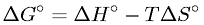Equations > Chemistry > Thermochemistry > Gibb's Free Energy Change in Terms of Enthalpy, Absolute Temperature, and Entropy

Gibb's Free Energy Change in Terms of Enthalpy, Absolute Temperature, and EntropyLatex Code:

MathML Code:

 $\Delta {G}^{\circ }=\Delta {H}^{\circ }-T\Delta {S}^{\circ }$

MathType 5.0: